# Algebra 1 - Functions

## Introduction

• Functions and relations are useful representations of many real-life situations. Functions are everywhere. From a larger perspective, what makes mathematics useful to the world is that different types of functions can model situations encountered by many people.
• In the field of Calculus, functions are building blocks of concepts because it studies differentiable functions from real numbers to real numbers.
• The function is a rule of correspondence between two nonempty sets, such that, to each element of the first set called domain, there corresponds one and only one element of the second set called range.

## Types of Functions and Key Features of Functions using Interval Notation

• Linear Function is a function of the form  where $a\ne 0$. We can use the rule $f\left(x\right)=ax+b$ to guide us in deriving the equation of the linear function when the table of values is given. The slope of the line represented by a linear function can be computed using the formula $m=\frac{{y}_{2}-{y}_{1}}{{x}_{2}-{x}_{1}}$.
• We use the point-slope form $y-{y}_{1}=m\left(x-{x}_{1}\right)$ to derive the equation of the linear function when the graph and table of values are given.
• Quadratic Function is a function of the form $f\left(x\right)=a{x}^{2}+bx+c$ where $a\ne 0$.
• Polynomial Function of degree $n$ is a function in the form ${a}_{n}{x}^{n}+{a}_{n-1}{x}^{n-1}+{a}_{n-1}{x}^{n-2}+...+{a}_{1}x+{a}_{0}$ such that $n\ne 0$.
• Piecewise Function is a function where there are different definitions for the different x values of its domain.
• Rational Function is a function of the form $f\left(x\right)=\frac{p\left(x\right)}{q\left(x\right)}$ where $p\left(x\right)$ and $q\left(x\right)$ are polynomials and $q\left(x\right)\ne 0$. Its parent function is defined by $f\left(x\right)=\frac{1}{x}$.
• Absolute Value Function is a function for all real numbers $x$, it is written in the form .
• Square Root Function is a function of the form $f\left(x\right)=\sqrt{x}$ where $x\ge 0$
• Cube Root Function is a function of the form $f\left(x\right)=\sqrt{x}$ where $x\in \mathrm{ℝ}$.
• Study the graph of $f\left(x\right)={x}^{2}-4x-5$ below with its key properties: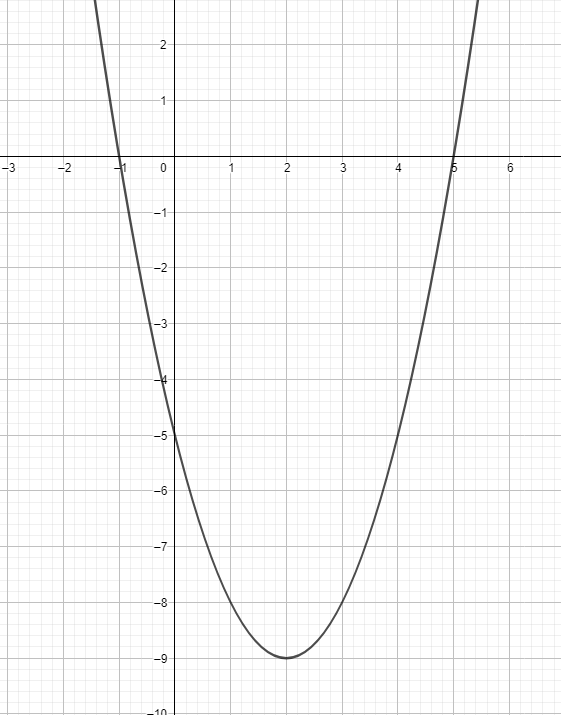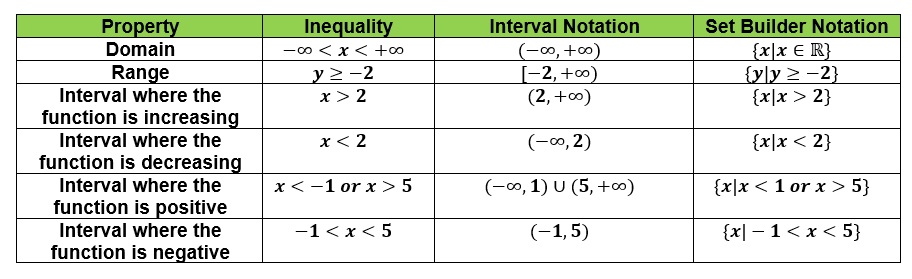## Transformations from the Graphs of Functions

• Here is the summary of transformations of function in terms of their graphs.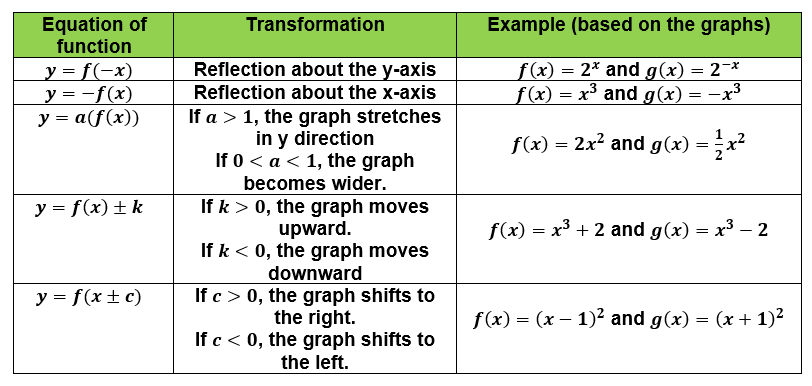## Absolute Value Functions and Piecewise Functions

Absolute Value Functions

• The properties of an absolute value function are the same as that of quadratic functions. The only difference is that the graph of absolute value function is V-shape.
• The parent function is $f\left(x\right)=\left|x\right|$.
• An absolute value function with transformation is expressed as  (Vertex form).
• Study the graphs of and $h\left(x\right)=\left|x+1\right|-3$ below.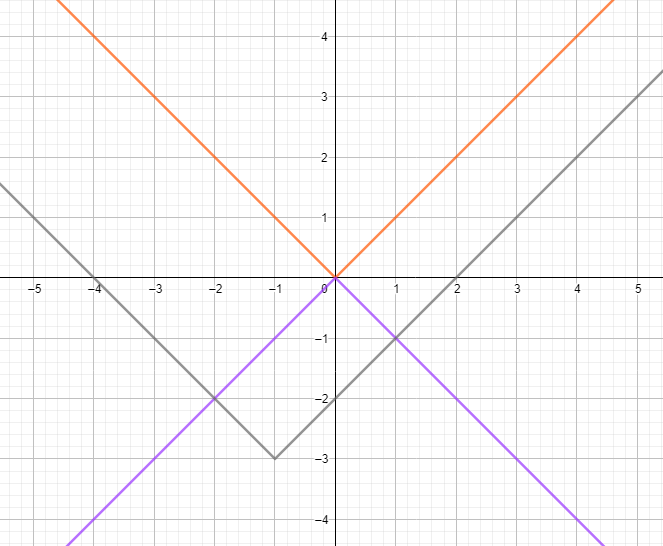Piecewise Functions

• These functions are defined by multiple sub-functions, where each applies to a certain interval of the main function's domain.
• This function is split into two or more functions whose domain is split into two or more mutually exclusive intervals.
• Consider the example of .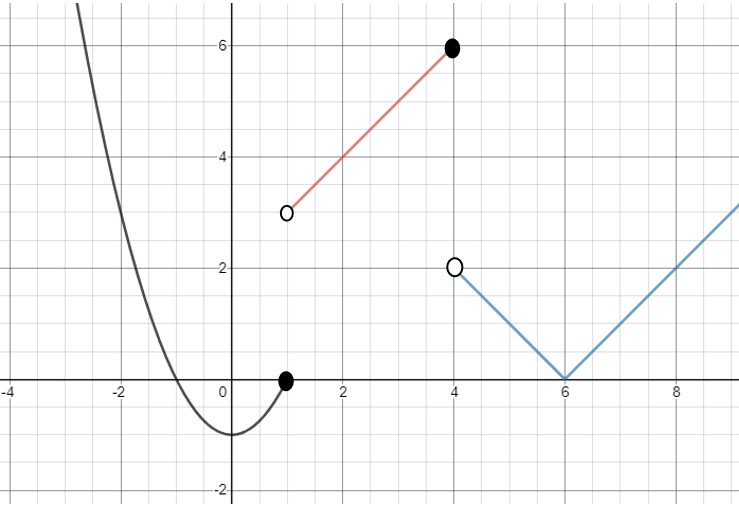• Consider the example . Evaluate $f\left(-4\right)$

Solution:

Evaluate $f\left(-4\right)$ means we need to find the function value when $x=-4$.

Since $x=-4$ belongs to the domain of $f\left(x\right)={x}^{3}+2$, we use this function.

$f\left(-4\right)={\left(-4\right)}^{3}+2$

$f\left(-4\right)=-62$

## Representing Real-Life Situations using Functions

• There are many practical applications of functions - science, business, work rate, number-related, geometry, and measurement, to name a few.
• The key concept of solving a word problem is to model the real-life situation by an appropriate function.
• One classical approach to solving a problem is Polya's Four Steps in Problem Solving.
• To represent real-life situations and solve for the unknown involving functions, we can follow the following steps:
1. Investigate the problem carefully.
2. Determine the independent and dependent variables to be represented by $x$ and $f\left(x\right)$, respectively.
3. Formulate the model (equation of the function).
4. Apply the model to find the unknown quantities required in the problem.

## Cheat Sheet

• In linear functions, the parent function is $f\left(x\right)=x$, and the equation describing transformations is $f\left(x\right)=a\left(x±c\right)±k$ where $a\ne 0$.
• In quadratic functions, the parent function is $f\left(x\right)={x}^{2}$, and the equation describing transformations is $f\left(x\right)=a{\left(x±h\right)}^{2}±k$ where $a\ne 0$.
• In exponential functions, the parent function is $f\left(x\right)={b}^{x}$, and the equation describing transformations is $f\left(x\right)=a{\left(b\right)}^{x±c}±k$ where $a\ne 0$.
• In absolute value functions, the parent function is $f\left(x\right)=\left|x\right|$, and the equation describing transformation is $f\left(x\right)=a\left|x±h\right|±k$ where $a\ne 0$.
• In cubic functions, the parent function is $f\left(x\right)={x}^{3}$, and the equation describing transformation is $f\left(x\right)=a{\left(x±c\right)}^{3}±k$ where $a\ne 0$.
• In square root function, the parent function is $f\left(x\right)=\sqrt{x}$, and the equation describing transformation is $f\left(x\right)=a\sqrt{x±h}±k$, where $a\ne 0$.

## Blunder Areas

• The square root function must not be written in the form $y=±\sqrt{x}$.
• Absolute value and quadratic functions are only different in terms of the shape of their graph.
• The domains of sub-functions in a piecewise function must be mutually exclusive.
• To determine whether a function is increasing or decreasing has a broader background and explanation in Differential Calculus.
• We can derive the equation of a function when the table of values is given.
• Always be careful in understanding the difference between $y=-f\left(x\right)$ and $y=f\left(-x\right)$ in terms of reflection.
• Be mindful of the signs involved when dealing with the horizontal translation of the graph of functions. For instance, $f\left(x\right)=2{\left(x-3\right)}^{2}$ is not the same with $f\left(x\right)=2{\left(x+3\right)}^{2}$.